2017-07-27 17:53:59 t5131828 阅读数 266
• ###### 5天搞定深度学习进阶系列教程

这门课程是“深度学习入门系列培训教程”的后续内容，深度学习进阶系列培训课程概况： 在深度学习入门系列的课程中，我们基本掌握了一些基础知识，在这门课程中我们将进一步学习深度学习原理、核心内容。包括深度置信网络DBN学习，卷积神经网络CNN入门到精通，深度残差网络RES和长短时记忆网络LSTM的构建以及使用。 后一部分我们也会稍微讲解一些人工智能前沿的发展强化学习RL，迁移学习TL和生成式对抗网络GAN。

3501 人正在学习 去看看 覃秉丰
5天搞定深度学习进阶系列教程—2222人已学习这门课程是“深度学习入门系列培训教程”的后续内容，深度学习进阶系列培训课程概况： 在深度学习入门系列的课程中，我们基本掌握了一些基础知识，在这门课程中我们将进一步学习深度学习原理、核心内容。包括深度置信网络DBN学习，卷积神经网络CNN入门到精通，深度残差网络RES和长短时记忆网络LSTM的构建以及使用。 后一部分我们也会稍微讲解一些人工智能前沿的发展强化学习RL，迁移学习TL和生成式对抗网络GAN。

理解并学会深度学习中最热门最核心的深层网络。

覃秉丰更多讲师课程
机器学习，深度学习神经网络领域多年开发研究经验，精通算法原理与编程实践。曾完成过多项图像识别，目标识别，语音识别的实际项目，经验丰富。关注深度学习领域各种开源项目，如TensorFlow，Caffe，Torch等。喜欢理论与实践相结合的教学风格，课程编排由浅入深，体系清晰完整。

第1章:深度置信网络DBN-DNN
1.课程介绍，推荐系统算法介绍  18:36
2.受限玻尔兹曼机在推荐系统中的应用  19:28
3.深度置信网络DBN-DNN  19:08
第2章:卷积神经网络CNN
1.卷积神经网络CNN  20:00
2.手写体识别网络LeNET-5结构分析  28:58
3.ImageNet介绍  8:33
4.ILSVRC12图像识别比赛冠军AlexNet  38:57
5.GPU及TPU介绍  9:04
第3章:152层深度残差网络RES
1.深度残差网络RES（论文讲解）  43:07
2.批量正则化Batch Normalization  21:03
3.深度残差网络进一步研究（论文讲解）  14:00
第4章:长短时记忆网络LSTM
1.递归神经网络RNN  11:03
2.长短时记忆网络LSTM  19:14
第5章:人工智能前沿方向
1.强化学习RL  17:37
2.迁移学习TL  8:35
3.生成式对抗网络GAN  13:07

2017-07-27 17:54:10 t5131828 阅读数 316
• ###### 5天搞定深度学习进阶系列教程

这门课程是“深度学习入门系列培训教程”的后续内容，深度学习进阶系列培训课程概况： 在深度学习入门系列的课程中，我们基本掌握了一些基础知识，在这门课程中我们将进一步学习深度学习原理、核心内容。包括深度置信网络DBN学习，卷积神经网络CNN入门到精通，深度残差网络RES和长短时记忆网络LSTM的构建以及使用。 后一部分我们也会稍微讲解一些人工智能前沿的发展强化学习RL，迁移学习TL和生成式对抗网络GAN。

3501 人正在学习 去看看 覃秉丰
5天搞定深度学习框架-Caffe入门系列—8916人已学习了解caffe基本框架，以及文件配置。学会绘制网络结构图，特征平面图，loss曲线图和accuracy曲线图。学会使用caffe训练模型的完整流程，并学会训练自己的模型。

覃秉丰更多讲师课程
机器学习，深度学习神经网络领域多年开发研究经验，精通算法原理与编程实践。曾完成过多项图像识别，目标识别，语音识别的实际项目，经验丰富。关注深度学习领域各种开源项目，如TensorFlow，Caffe，Torch等。喜欢理论与实践相结合的教学风格，课程编排由浅入深，体系清晰完整。

1.Caffe介绍  12:18
2.Caffe在windows下的安装编译  8:25
3.Caffe快速上手-mnist数据集分类(一)  20:27
4.Caffe快速上手-mnist数据集分类(二)  20:26
5.Caffe文件详解  44:17
6.各种优化器的介绍  25:30
7.Caffe的python接口安装，以及模型可视化  28:33
8.Caffe特征图可视化以及学习曲线可视化  45:14
11.Caffe在windows下GPU版本的安装  14:53
12.使用自己设计的网络训练自己的图像识别模型(一)  26:08
13.使用自己设计的网络训练自己的图像识别模型(二)  39:16
14.迁移学习-Finetune  27:51
15.Snapshot以及课程总结  8:27

2018-04-06 07:53:25 xundh 阅读数 1432
• ###### 5天搞定深度学习进阶系列教程

这门课程是“深度学习入门系列培训教程”的后续内容，深度学习进阶系列培训课程概况： 在深度学习入门系列的课程中，我们基本掌握了一些基础知识，在这门课程中我们将进一步学习深度学习原理、核心内容。包括深度置信网络DBN学习，卷积神经网络CNN入门到精通，深度残差网络RES和长短时记忆网络LSTM的构建以及使用。 后一部分我们也会稍微讲解一些人工智能前沿的发展强化学习RL，迁移学习TL和生成式对抗网络GAN。

3501 人正在学习 去看看 覃秉丰

# 概念

$r={d}_{j}-{o}_{j}$

$f\left({W}_{j}^{T}X\right)=sgn\left({W}_{j}^{T}X\right)=\left\{\begin{array}{ll}1,& {W}_{j}^{T}X\ge 0\\ 0,& {W}_{j}^{T}<0\end{array}$

$W$$W$代表矩阵， $w$$w$代表一个值。

$\mathrm{\Delta }{W}_{j}=\eta \left[{d}_{j}-sgn\left({W}_{j}^{T}X\right)\right]X$

$\mathrm{\Delta }{w}_{ij}=\eta \left[{d}_{j}-sgn\left({W}_{j}^{T}X\right)\right]{x}_{i}i=0,1,...,n$

• $\eta$$\eta$表示学习率，（$0<\eta \le 1$$0< \eta \le1$
• 两次迭代之间的权值变化已经很小
• 设定最大迭代次数，当迭代超过最大次数就停止。

$\mathrm{\Delta }{W}_{j}=±2\eta X$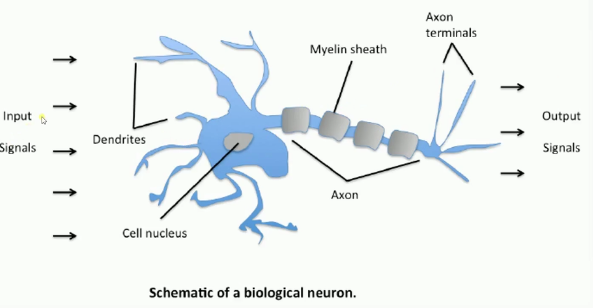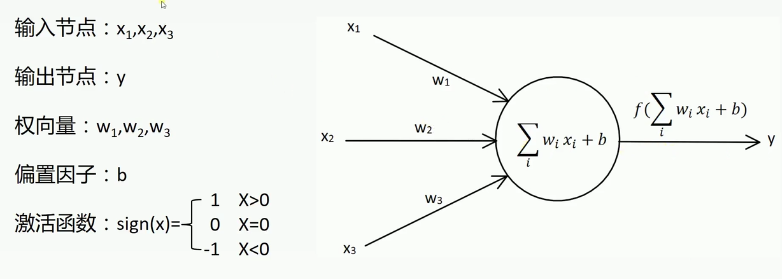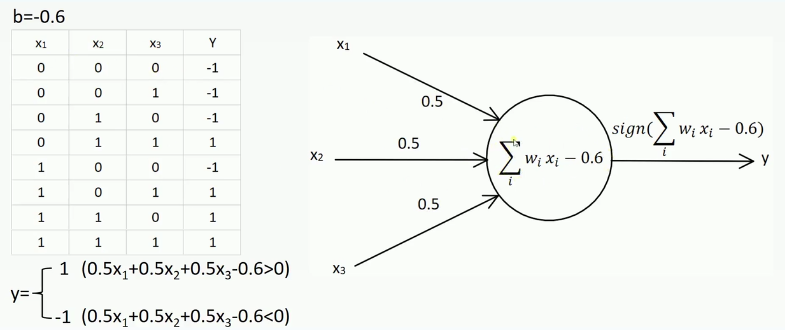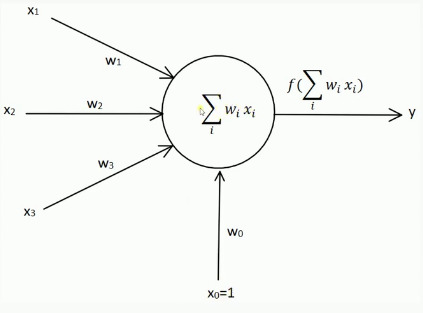2018-08-10 12:21:13 iamsrc 阅读数 2014
• ###### 5天搞定深度学习进阶系列教程

这门课程是“深度学习入门系列培训教程”的后续内容，深度学习进阶系列培训课程概况： 在深度学习入门系列的课程中，我们基本掌握了一些基础知识，在这门课程中我们将进一步学习深度学习原理、核心内容。包括深度置信网络DBN学习，卷积神经网络CNN入门到精通，深度残差网络RES和长短时记忆网络LSTM的构建以及使用。 后一部分我们也会稍微讲解一些人工智能前沿的发展强化学习RL，迁移学习TL和生成式对抗网络GAN。

3501 人正在学习 去看看 覃秉丰

# 第一天 数据处理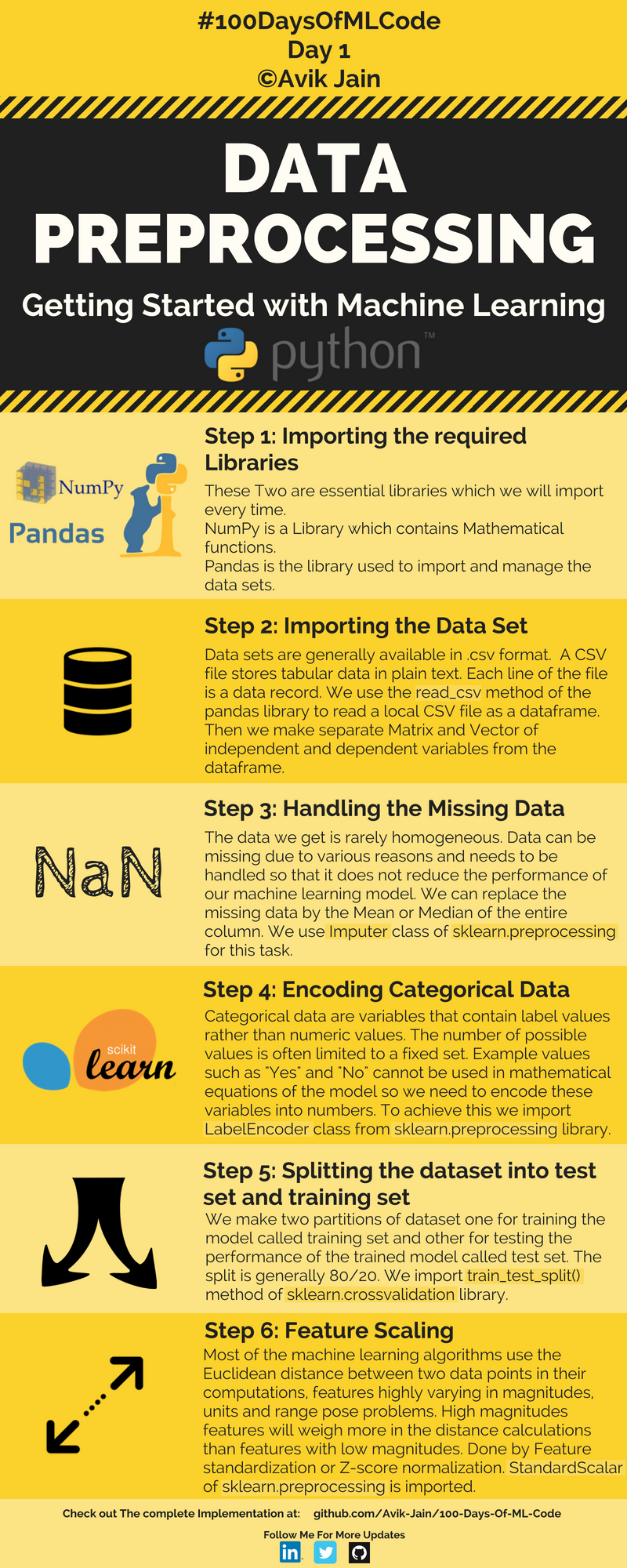## 第一步：导入必须的包

these two are essential libraries which we will import every time.

Numpy is a libray which contains Mathematical function

Numpy库包含了数学处理函数

pandas is the library used to import and manage the data sets

pandas 是用来导入和管理数据集的库

## 第二步：导入数据集

data sets are generally available in .csv format

a CSV file stores tabular data in plaintext

csv 文件以明文存储表格数据

each line of the file is a data record

we use the read_csv method of the pandas library to read a local csv file as a dataframe

then we make separate Matrix and Vector of independent and dependent variables from the dataframe

## 第三步： 处理丢失数据

the data we get is rarely homogeneous

data can be missing due to various reasons and needs to be handled so that it does not reduce the performance of our machine learning model

We can replace the missing data by the Median of the entire column

we use imputer class of sklearn.preprocessing for this taske

## 第四步：编码分类的数据

categorical data are variables that contain lable values rather than numeric values.

the number of possible values is often limited to a fixed set.

Example values such as “yes” and “No” cannot be used in mathematical equations of the model so we need to encode these variables into numbers

To achieve this we import LableEncoder class from sklearn.preprocessing library.

## 第五步：把数据集分为测试集和训练集

we make two partitions of dataset one for training the model called training set and other for testing the performance of the trained model called test set

the split is generally 80/20

we importing train_test_split() method of sklearn.crossvalidation library

## 第六步：特征缩放

most of machine learning algorithms use the Euclidean distance between two data points in their computations,features highly varying in magnitudes, units and range pose problems.

High magnitudes features will weigh more in the distance calculations than features with low magnitudes.

Done by feature standardization or Z-score normalization

StandardScalar of sklearn.preprocessing is imported

## 代码如下

### Step 1: Importing the libraries 导入库

import numpy as np
import pandas as pd

### Step 2: Importing dataset 导入数据集

dataset = pd.read_csv('Data.csv')
X = dataset.iloc[ : , :-1].values
Y = dataset.iloc[ : , 3].values

### Step 3: Handling the missing data 处理丢失数据

from sklearn.preprocessing import Imputer
imputer = Imputer(missing_values = "NaN", strategy = "mean", axis = 0)
imputer = imputer.fit(X[ : , 1:3])
X[ : , 1:3] = imputer.transform(X[ : , 1:3])

### Step 4: Encoding categorical data 编码分类的数据

from sklearn.preprocessing import LabelEncoder, OneHotEncoder
labelencoder_X = LabelEncoder()
X[ : , 0] = labelencoder_X.fit_transform(X[ : , 0])

### Creating a dummy variable 穿件中间变量

onehotencoder = OneHotEncoder(categorical_features = )
X = onehotencoder.fit_transform(X).toarray()
labelencoder_Y = LabelEncoder()
Y =  labelencoder_Y.fit_transform(Y)

### 数据集分割为训练集和测试集

from sklearn.cross_validation import train_test_split
X_train, X_test, Y_train, Y_test = train_test_split( X , Y , test_size = 0.2, random_state = 0)

### Step 6: Feature Scaling 特征缩放

from sklearn.preprocessing import StandardScaler
sc_X = StandardScaler()
X_train = sc_X.fit_transform(X_train)
X_test = sc_X.fit_transform(X_test)

## 杂谈：

sklearn是机器学习中一个常用的python第三方模块，网址：http://scikit-learn.org/stable/index.html ，里面对一些常用的机器学习方法进行了封装，在进行机器学习任务时，并不需要每个人都实现所有的算法，只需要简单的调用sklearn里的模块就可以实现大多数机器学习任务。